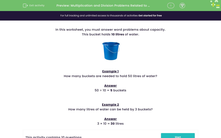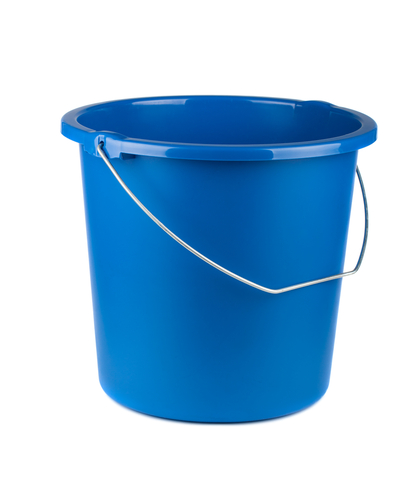# Solve Multiplication and Division Problems Related to Capacity

In this worksheet, students will answer simple multiplication and division questions related to the capacity of a 10-litre bucket.Key stage:  KS 1

Curriculum topic:   Number: Multiplication and Division

Curriculum subtopic:   Solve Problems Using Multiplication/Division

Difficulty level:#### Worksheet Overview

This bucket holds 10 litres of water.Example 1

How many buckets are needed to hold 50 litres of water?

10 x ? = 50

One way to find the answer:

1 bucket = 10 litres

2 buckets = 20 litres

3 buckets = 30 litres

4 buckets = 40 litres

5 buckets = 50 litres

A quicker way is to  use division to find the answer:

50 ÷ 10 = 5 buckets

Example 2

How many litres of water can be held by 3 buckets?Again, 1 bucket = 10 litres

2 buckets = 20 litres

3 buckets = 30 litres

It is quicker to use multiplication:

3 × 10 = 30 litres

Let's have a go at some questions now.### What is EdPlace?

We're your National Curriculum aligned online education content provider helping each child succeed in English, maths and science from year 1 to GCSE. With an EdPlace account you’ll be able to track and measure progress, helping each child achieve their best. We build confidence and attainment by personalising each child’s learning at a level that suits them.

Get started